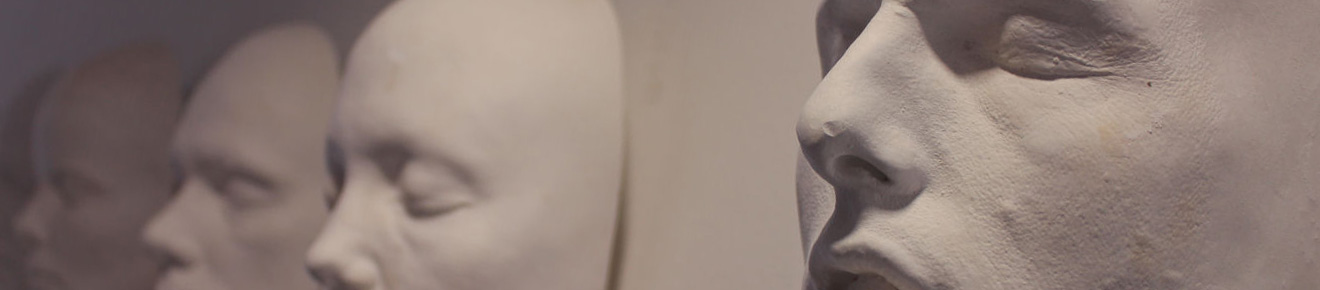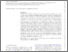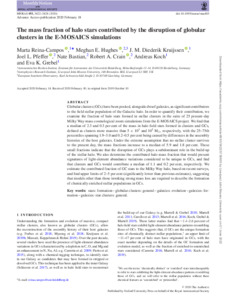# The mass fraction of halo stars contributed by the disruption of globular clusters in the E-MOSAICS simulations

Reina-Campos, M, Hughes, ME, Kruijssen, JMD, Pfeffer, JL, Bastian, N, Crain, RA, Koch, A and Grebel, EK (2020) The mass fraction of halo stars contributed by the disruption of globular clusters in the E-MOSAICS simulations. Monthly Notices of the Royal Astronomical Society, 493 (3). pp. 3422-3428. ISSN 0035-8711Preview
Text
staa483.pdf - Published Version

Globular clusters (GCs) have been posited, alongside dwarf galaxies, as significant contributors to the field stellar population of the Galactic halo. In order to quantify their contribution, we examine the fraction of halo stars formed in stellar clusters in the suite of 25 present-day Milky Way-mass cosmological zoom simulations from the E-MOSAICS project. We find that a median of $2.3$ and $0.3$ per cent of the mass in halo field stars formed in clusters and GCs, defined as clusters more massive than $5\times 10^3$ and $10^5~M_{\odot}$, respectively, with the $25$-$75$th percentiles spanning $1.9$-$3.0$ and $0.2$-$0.5$ per cent being caused by differences in the assembly histories of the host galaxies. Under the extreme assumption that no stellar cluster survives to the present day, the mass fractions increase to a median of $5.9$ and $1.8$ per cent. These small fractions indicate that the disruption of GCs plays a sub-dominant role in the build-up of the stellar halo. We also determine the contributed halo mass fraction that would present signatures of light-element abundance variations considered to be unique to GCs, and find that clusters and GCs would contribute a median of $1.1$ and $0.2$ per cent, respectively. We estimate the contributed fraction of GC stars to the Milky Way halo, based on recent surveys, and find upper limits of $2$-$5$ per cent (significantly lower than previous estimates), suggesting that models other than those invoking strong mass loss are required to describe the formation of chemically enriched stellar populations in GCs.View Item